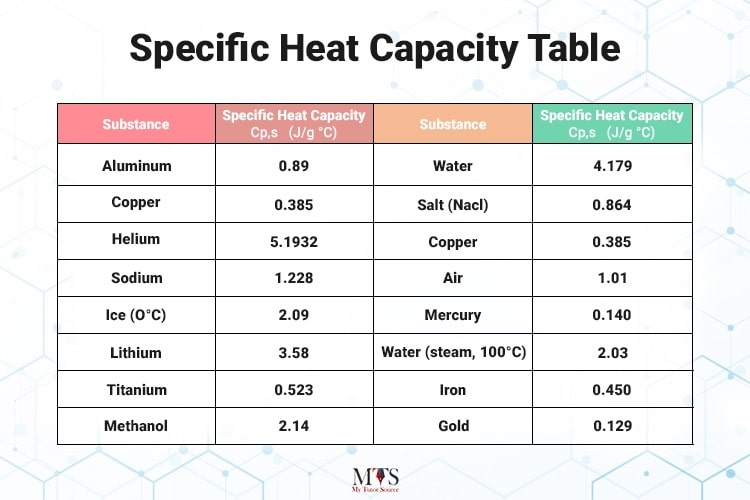# Learn About Specific Heat Capacity in Less Than 10 Minutes!

• Published On:Chemistry has always been a difficult subject for most students. It is filled with complex formulae, complicated molecule structures, and many super-confusing concepts.

Truth be told, chemistry is not hard to learn if you have a good instructor or an online chemistry tutor on your side and if you read the right books plus the contents.

Here in this blog, we’ve explained everything about specific heat capacity in easy words. Just to help you get good scores in your Chemistry exam.

So, let’s move on…

## Quick Definition!

“The amount of heat energy absorbed by a substance to raise its temperature per unit mass is defined as specific heat capacity.”

Let’s say we’ve two substances: substance X and substance Y. The temperature of substance X is less than the temperature of substance Y. In this case, the heat energy of system Y will flow into system X via the surrounding. System A's molecule will keep receiving the energy heat to increase the temperature.

Now read this carefully … the energy absorbed per unit mass by the system X to raise its temperature per unit is called specific heat capacity. This temperature is referred to as “c.”

## Important Points to Remember:

• Heat always transfers from a warmer substance to a cooler one.
• The heat absorbed by the cooler substance is always equal to the amount of heat supplied by the warmer substance.
• Every substance has its own specific heat capacity.

## The SI Unit of Specific Heat Capacity is  “c”

Scientifically, the SI unit of specific heat capacity is J kg - 1 K - 1. In easy words, “c” is the heat required to raise 1 gram of any substance to 1 kelvin temperature. Scientists also write the SI unit of the specific heat capacity as “J/kg·K”.

## How to Calculate Specific Heat Capacity?

Here is the formula used for calculating specific heat capacity.
Q = C m ∆t

Where;
• Q = amount of heat absorbed by a substance
• m = mass of the body
• ∆t = Rise in temperature
• C = Specific heat capacity of a substance

## Specific Heat Capacity of Different Substances

When the same amount of heat is given to two substances of the same masses, you’ll notice the rise in temperature of each substance is unique. This is why it takes longer for water to heat up on a stove than it does for iron at the same room temperature.
Here is the table below that highlights the specific heat capacity of different products:### Is specific heat capacity intensive or extensive?

Justification: Specific heat capacity is intensive, which means that it doesn’t depend on the amount of matter in the substance. Further, its units of energy are always per mass per degree.

### Why does water have a high Specific heat capacity?

Quick Answer: Because of the Hydrogen Bonding

Justification: When the water is heated, hydrogen bonding is formed, which as a result releases heat. This is the reason why water has high specific heat capacity!

### Explain the difference between heat capacity and specific heat capacity.

Quick Answer: Heat Capacity (to raise temp. by one unit); Specific Heat Capacity (to raise temp. by one unit per mass)

Justification: Heat capacity is defined as the potential of a substance to absorb energy for raising its temperature to one unit. On the other hand, specific heat capacity is the potential of any substance to receive energy to raise its temperature by one unit per mass.

### Find Top Tutors in Your AreaAustin has 10+ years of experience in teaching. He has researched on thousands of students-related topics, issues, and concerns. You will often find him writing about the common concerns of students, their nutrition, and what is beneficial for their academics and health both.